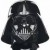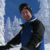# Probability of an Average for a Group Based on Individual Value Distribution

Six Sigma – iSixSigma Forums General Forums Tools & Templates Probability of an Average for a Group Based on Individual Value Distribution

Viewing 6 posts - 1 through 6 (of 6 total)
• Author
Posts
• #240205Laura Stolar
Participant

I have a normal distribution for a coating weight per part of 1 gram/m2 and a standard deviation of 0.01 g/m2. Our customer would like to know the chance of getting 100 parts that average 0.95 g/m2. Not whether every part is 0.95, but that the average of the 100 parts is 0.95 g/m2. It seems that if the probability of one part being 0.95 is 0.287 ppm, the likelihood of getting 100 parts with that average is much smaller, but would it actually be 0.287^100? I would expect that would be the likelihood of every part being 0.95 rather than getting 100 parts which average to 0.95. The standard deviation is the long term standard deviation over a long period and we have SPC to alert us of any special causes, so we should be robust to any unlikely run of many low parts.

0
#240223Ken Feldman
Participant

@lausto @RobertButler While the problem you are asking is an interesting stat question I would wonder why a customer would even ask such a question. What are the specs for the part coating weight? I would assume that your customer would want all parts to be in spec not the average of parts to be in spec. You can have parts equally varying from .94 to .96 and get an average of .95. Or you can have half the parts with 0 coating and half at 1.90. I would defer the stat calculation to my learned associate Robert Butler.

0
#240226Laura Stolar
Participant

@Darth, @RobertButler
All of the parts need to be in spec, but they are also wondering about at a stack up tolerance. If they stack 100 of these parts together, the final height should be on average the thickness of a 1 gsm coating x 100 (plus substrates). They are wondering the chance of getting a short height because many of the individual parts were on the low end of the specification.

I have simply assumed a FIFO of the parts and found the average for the last 15 groups of 100 at this point, but I was thinking there may be a way to transform an individual distribution to a grouped distribution.

0
#240269Robert Butler
Participant

I think you will need to provide some additional details.

1. In your first post you are talking about weight and in your second post you are talking about height – which is it?
2. In your first post you will need to clarify what you mean by “I have a normal distribution for a coating weight per part of 1 gram/m2 and a standard deviation of 0.01 g/m2.”
a. As written it could be taken to mean you have a normal distribution with an average of 1 gram/m2 and a long term population sample deviation of .01 gm/m2 or it could mean you have a part that is 1 gram/m2 which comes from a population that has a population standard deviation of .01 gm/m2. There is also the question of the standard deviation – is it a long term population standard deviation or is it a long term standard deviation of the mean (in which case we will need to know the sample size used to generate the estimate of the standard deviation of the mean).

If we assume the 1 gm/m2 represents the long term population mean and if we assume the standard deviation is an estimate of the population mean then the chances of getting a part, never mind a random collection of 100 parts, with an average value of .95 is nil because 3 x the standard deviation is only going to span a range of .97 to 1.03.

If you can provide more information perhaps I or someone else might be able to offer some suggestions.

0
#240274Ken Feldman
Participant

@RobertButler Hi Robert. I tossed this thread to you because I missed all your great responses. Thanks for jumping in. Hope all is well. Not much action or volume here any more like the old days. Except for you and Carnell.

0
#240300Chuck White
Participant

I agree with @rbutler — if the process average is actually 1.0 and the standard deviation is 0.01, then the probability is close enough to zero to not worry about it.

But if you want to calculate it out, I do need to clarify something else. Are you asking the probability of an n=100 sample average equal to 0.95 or less than 0.95? The probability of a sample average being an exact number is essentially zero no matter how close it is to the population average. In practice it would depend on your measurement resolution.

I am guessing you are really interested in the probability of a sample average less than (or <= if you prefer) 0.95. For that calculation, you need to use the standard error instead of the standard deviation. The standard error is the standard deviation of all possible sample means of a given sample size, and it is equal to the standard deviation divided by the square root of the sample size. In your case that would be 0.01 divided by the square root of 100 (10) = 0.001. So your hypothetical sample average is 50 standard errors (.05/.001) from the population mean, so you would need to find the probability for a z value of 50. I am not aware of any z table that goes anywhere near 50, so I’ll go back to my original statement that it is close enough to zero to never worry about.

0
Viewing 6 posts - 1 through 6 (of 6 total)

You must be logged in to reply to this topic.